How to use MAXIFS functionMAXIFS function is considered to be one of the advanced statistical functions available in Excel. This is a new function added to Excel, so only available in the latest version of Office (EXCEL 2016, Excel Online and latest mobile excel versions).

The MAXIFS function is used to return the maximum value of a selected data range, considering a set of pre-specified conditions or criteria.

The syntax used is explained below:

MAXIFS(max_range, criteria_range1, criteria1, [criteria_range2, criteria2], ...)

max_range:                      The range of cells, from which the maximum value to be identified and returned. (Required)

criteria_range1:                The range of cells to consider with the criteria 1. (Required)

criteria1:                          The criteria for the criteria range 1, this can be specified in the form of a numerical value, expression, text. The criteria will define which cells to be considered to find the maximum. (Required)

criteria_range2:               An additional range of cells, to consider with criteria 2. Use of criteria 2 onwards is optional.

criteria2:                         The criteria for the criteria range 2, 126 number [range/criteria] pairs can be used.

Note: the shape and size of the max range and criteria_range1,2,3… must be equal.  If not an error value would be returned (#VALUE! error)

Example #1:

Consider the following data set, and think you want to find the maximum amount of Apple sales in a month.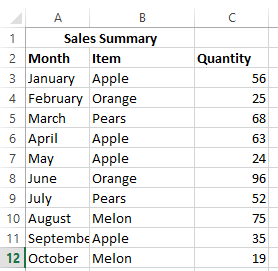You can use the following syntax arguments to easily find the max. apple sales.

=MAXIFS(C3:C12,B3:B12,”Apple”)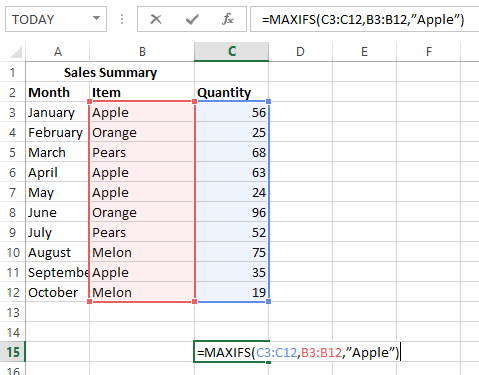The Answer will be 63.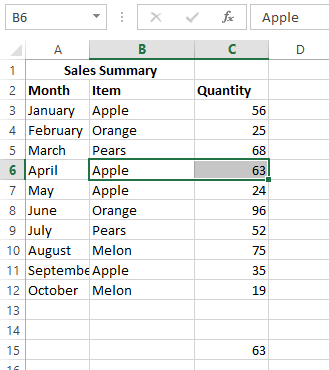Example #2:

The following example will show how multiple criteria used with MAXIFS function. The number of max. apple sales to be found with rejects less than 10.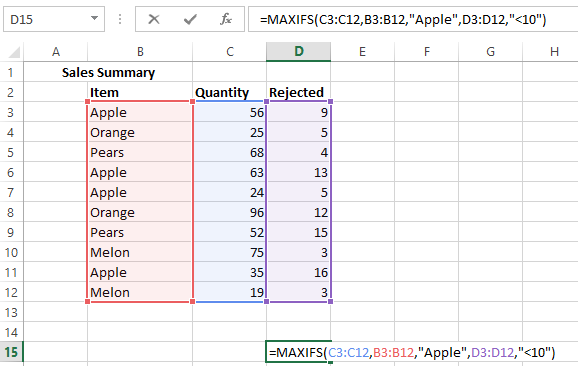The Answer will be 56.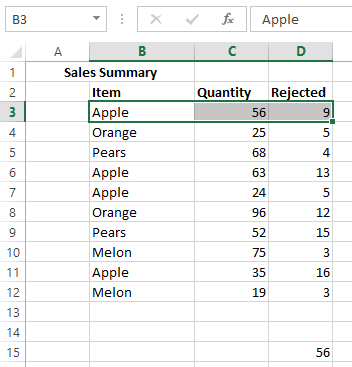Note: If the size and the shape of the max_range and criteria_range are different, then it would gives an error as explained in the following example.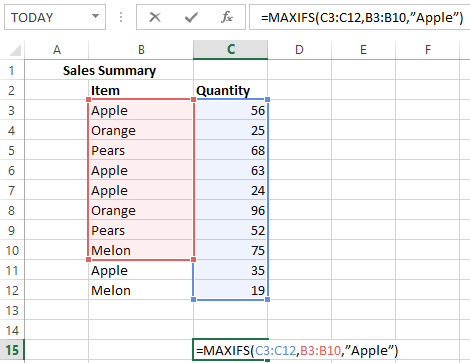The result will be #VALUE!.

If there is no cell match with criteria provided, then the result will be 0.